×#### Thank you for registering.

One of our academic counsellors will contact you within 1 working day.

Click to Chat

1800-1023-196

+91-120-4616500

CART 0

• 0

MY CART (5)

Use Coupon: CART20 and get 20% off on all online Study Material

ITEM
DETAILS
MRP
DISCOUNT
FINAL PRICE
Total Price: Rs.

There are no items in this cart.
Continue Shopping• Complete JEE Main/Advanced Course and Test Series
• OFFERED PRICE: Rs. 15,900
• View Details

```Revision Notes on Mensuration

Mensuration

It is all about the measurement of area, perimeter and volume of the plane and solid figures.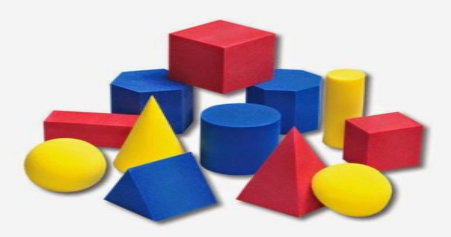Area

The surface covered by the border line of the figure is the area of the plain shape.

Unit of the area is square if the length unit.

Perimeter

The perimeter is the length of the boundary of the plane shape.

The unit of the perimeter is same as the length unit.The green part is the area of the square and the distance all the way around the outside is the perimeter.

Area and Perimeter of Some 2D Shapes

Shape
Image
Area
Perimeter

Square(Side)2
4 × Side

Rectangle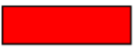Triangle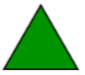(1/2) × Base × Height
where, a, b and c are the three sides of the triangle)
a + b + c

Parallelogram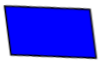Base × Height

Circleπr2
2πr
Where, r = radius of the circle

Area of Trapezium

A trapezium is a quadrilateral whose two sides are parallel. And if its non-parallel sides are equal then it is said to be an isosceles trapezium.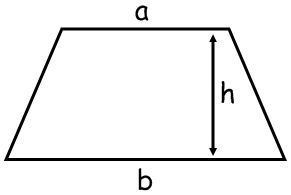Area of Trapezium can be found,

1. By Splitting the figure

One way to find the Area of trapezium is to divide it into two or three plane figures and then find the area.In the trapezium ABCD,

It can be divided into two parts i.e. a rectangle and a triangle.

Area of ABCD = Area of ABED + Area of DEC

2. By using formula

Another way is to calculate the area by using formula.Area of trapezium is half of the product of the summation of the parallel sides and the perpendicular distance between them.

Example

Find the area of the trapezium whose parallel sides are 6 cm and 16 cm, with a height of 5 cm. Calculate the area using both the methods.Solution:Splitting the trapezium we get –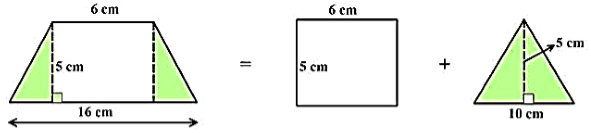Area of the trapezium = Area of rectangle + Area of a triangle

= (6 x 5) + (1/2) x 5 x 10

= 30 + 25

= 55 cm2

Remark: We should use the formula most of the time if possible as it is the quick and easy method.To find the area of any quadrilateral we can divide it into two triangles and then the area can be easily calculated by calculating the area of both the triangles separately.

Area of ABCD = Area of ∆ABC + Area of ∆ACD

= (1/2) × AC × h1 + (1/2) × AC× h2

The formula for the Area of a General QuadrilateralWhere h1 and h2 are the height of both the triangles and d is the length of common diagonal i.e.AC.

Example

Find the area of quadrilateral ABCD.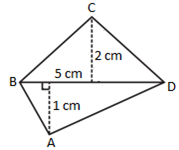Solution:

BD is the common diagonal so d = 5 cm.

Height of the two triangles are h1 = 2 cm and h2 = 1 cm.A rhombus is a quadrilateral with all the sides are equal and parallel but not the right angle. Its two diagonals are the perpendicular bisector to each other.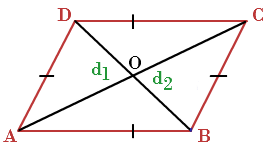In this also we can split the rhombus into two triangles and can find the area of rhombus easily.

Formula of Area of RhombusArea of rhombus is half of the product of its two diagonals.

Area of a Polygon

There is no particular formula for the area of the polygon so we need to divide it in a possible number of figures like a triangle, rectangle, trapezium and so on. By adding the area of all the split figures we will get the area of the required polygon.

Example

Find the area of the given octagon.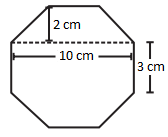Solution:

We can divide the given octagon into three parts.Two trapezium A and B and one rectangle shown by part B.

Two trapezium A and B and one rectangle shown by part B.
Area of A = Area of B = (1/2) × (a + b) × h

= (1/2) x (10 + 3) × 2

= 13 cm2.

Area of B = Length x Breadth

= 10 x 3

= 30 cm2.

So, the area of Octagon = 2A + B

= 2 × 13 + 30

= 56 cm2.

Solid Shapes

The 3-dimensional shapes which occupy some space are called solid shapes. Example- Cube, Cylinder, Sphere etc.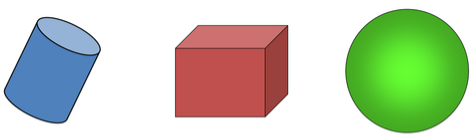Surface Area

If we draw the net of the solid shape then we can see it’s all the faces clearly and if we add the areas of all the faces then we get the total surface area of that solid shape. The unit of surface area is a square unit.

Lateral or Curved Surface Area

If we leave the top and bottom faces of the solid shape then the area of the rest of the figure is the lateral surface of the shape. The unit of lateral surface area is a square unit.

Surface Area of Cube, Cuboid and Cylinder

Name
Figure
Lateral or Curved Surface Area
Total Surface Area
Nomenclature

Cube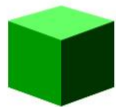4l2
6l2
l = Edge of the cube

Cuboid2h(l + b)
2(lb + bh + lh)
l = Length, b = Breadth, h = Height

Cylinder2πrh
2πr2+ 2πrh = 2πr(r + h)
r = Radius, h = Height

Volume

Volume is the space occupied by any solid figure i.e. the amount of capacity to carry something is the volume of that solid shape. The unit of volume is a cubic unit.

Volume of Cube, Cuboid and Cylinder

Name
Volume
Nomenclature

Cube
l3
l = Edge of the cube

Cuboid
lbh
l = Length, b = Breadth, h = Height

Cylinder
πr2h
r = Radius, h = Height

Example 1

There is a shoe box whose length, breadth and height is 9 cm, 3 cm and 4 cm respectively. Find the surface area and volume of the shoe box.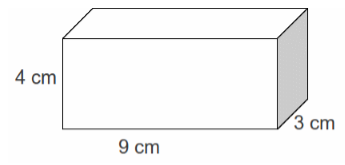Solution:

Given,

length = 9 cm

Height = 4 cm

Area of cuboid = 2(lb + bh + lh)

= 2(9 × 3 + 3×4 + 9 × 4)

= 2(27 + 12 + 36)

= 2(75)

= 150 cm2

Volume of cuboid = lbh

= 9 × 3 × 4

= 108 cm3

Example 2

If there is a cold drink can whose height is 7 cm and the radius of its round top is 3 cm then what will be the lateral surface area and volume of that cylinder? (π = 3.14)Solution:

Given,

Height = 7 cm

Lateral surface area of cylinder = 2πrh

= 2 × 3.14 × 3 × 7

= 131.88 cm2

Volume of cylinder = πr2h

= 3.14 × 3 × 3 × 7

= 197.82 cm3

Example 3

If there is a box of cube shape with the length of 4 cm then what will be the capacity of this box. Also, find the surface area of the box if it is open from the top.Solution:

Given, side = 4 cm

Capacity or volume of the box = s3

= 43 = 64 cm3

The total surface area of the box = 6s2

But, if the box is open from the top then the surface area will be total surface area minus the area of one face of the cube.

Surface Area = Total Surface Area - Area of one face

= 6s2 – s2

= 5s2 = 5 × 42

= 80 cm2

Volume and Capacity

Volume and capacity are one and the same thing.

Volume is the amount of space occupied by a shape.

Capacity is the quantity that a container can hold.

Capacity can be measured in form of liters.

We can see the relation between liter and cm3 as,

1 L = 1000 mL

1 mL = 1 cm3,

1 L = 1000 cm3.

Thus, 1 m3 = 1000000 cm3 = 1000 L.
```### Course Features

• 728 Video Lectures
• Revision Notes
• Previous Year Papers
• Mind Map
• Study Planner
• NCERT Solutions
• Discussion Forum
• Test paper with Video Solution